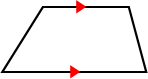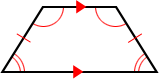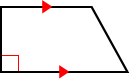# Trapezoid

A trapezoid is a quadrilateral with one pair of parallel sides. The figure below shows a few different types of trapezoids.

Note: Some define a trapezoid as a quadrilateral with at least one pair of parallel sides implying that it could contain two pairs of parallel sides, which would make it a parallelogram. For the sake of this article, we will define a trapezoid as a quadrilateral with only one pair of parallel sides.

## Sides of a trapezoid

The parallel sides of a trapezoid are referred to as its bases. The non-parallel sides are called legs. The height (or altitude) is the line segment used to measure the shortest distance between the two bases.

## Angles of a trapezoid

In a trapezoid, the pair of angles that share a common base are called base angles. For the trapezoids shown in the diagram below, ∠A and ∠D are base angles and ∠B and ∠C are base angles. The pair of angles next to a leg are supplementary: ∠A + ∠B = 180° and ∠C + ∠D = 180°.

## Midsegment of a trapezoid

The midsegment of a trapezoid is a line segment connecting the midpoint of its legs. A midsegment is parallel to the bases and has a length that is one-half the sum of the two bases.

In the figure above, midsegment EF divides legs AB and CD in half and## Area of a trapezoid

The area, A, of a trapezoid is one-half the product of the sum of its bases and its height.where h is the height and b1 and b2 are the base lengths.

## Trapezoid classifications

Trapezoids can be classified as scalene or isosceles based on the length of its legs. If the legs and base angles of a trapezoid are congruent, it is an isosceles trapezoid. Otherwise, it is a scalene trapezoid.

Scalene trapezoidIsosceles trapezoidLegs or base angles not congruent Congruent legs and base angles

Trapezoids can also be classified as right trapezoids or obtuse trapezoids based on its angles. If one of the legs is perpendicular to the bases, the trapezoid is a right trapezoid. Otherwise the trapezoid must contain two obtuse angles and is called an obtuse trapezoid.

Right trapezoidObtuse trapezoidOne leg is perpendicular to the bases. Two angles are obtuse.

## Isosceles trapezoids

An isosceles trapezoid is a special trapezoid with congruent legs and base angles. It has the following properties.

• The two diagonals of an isosceles trapezoid are congruent. They also form congruent triangles. In the isosceles trapezoid below, diagonals AC and BD are congruent. Since the legs of an isosceles trapezoid are congruent and the following pairs of triangles share a base, △ABD ≅ △DCA and △ABC ≅ △DCB by the Side-Side-Side postulate.
• The ratio of the segments making up the diagonals of a trapezoid are proportional. In the diagram above, AE = DE, BE = CE, and• An isosceles trapezoid has one line of symmetry: the altitude that bisects its bases. In the figure above, altitude FE bisects bases AD and BC. Reflecting isosceles trapezoid ABCE across FE preserves it, making FE a line of symmetry.